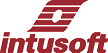Newsletter ListNewsletter Issue #77, July 2005

 Summer Update

Intusoft is excited to release its latest ICAP/4 8.x.11 software this summer. Over the past several months a dedicated technical team has put forth an unprecedented effort to provide valuable extensions to the existing ICAP/4 2450 build. The first of these capabilities stems from an intense modeling effort, assembled from the engineering community’s custom modeling requests. Many of the devices were very complex in nature. To the surprise of the requestors, the devices were successfully modeled, and with a very high level of accuracy. ICs such as sophisticated PWMs, regulators, and specialized operational amplifiers were amongst the list. Discrete devices included select Schottky diodes, an extensive collection of saturable magnetic cores to Magnetics Designer, and special power MOSFETs. Even a solar cell/panel was added to the ever-growing IsSpice4 model collection, which now totals more than 1,000 new full custom devices since the free service began a year ago. Several unique generic models have also been added, enabling the engineer to easily tweak inherent modeling parameters to create unique versions of ICAP/4’s newest op-amps, UJTs, SCRs, and power MOSFETs.

 In This Issue 1 2 Nonlinear Inductors in Magnetics Designer 3 4 Nonlinear Inductor Models 15 Summer Update (Continued)

The new generic models provide something no other SPICE tool furnishes – the ability to quickly create custom models from several different types of generic components, over and above the usual BJT, op-amp, diode, etc. The generic representations, of course, can also be used as is. Further, SpiceMod now accommodates new advanced N and P channel power MOSFETs. This adds to its existing provision of 15 different types of discrete component models, easily created from datasheet entry.

A major notable change is that ICAPS can now generates a new .PAR file to help users better understand and debug complex designs. This file consists of two sections. In the first section, all parameters defined in the top level of the design, and all default parameters defined in every subckt in the design, are listed with their values. In the second section, all passed parameters to every instance of every subckt in the design are listed along with the computed values of the parameters. This file is used in complex design (e.g. heirarchical drawings, multiple nesting of subckts, passed parameters with expressions) to confirm that the correct values are passed for every instance of a subckt.

 Nonlinear Inductors in Magnetics Designer

Many requests have been made for nonlinear core models, particularly for the SPICE models that come from the Magnetics Designer software. Magnetics Designer has some nonlinear parameters available internally, so that it’s possible to make an accurate model for many cores. The powdered cores, in particular, are valuable candidates for automated models. Adding the extra complexity to the model is generally unnecessary if a design doesn’t near saturation. The saturation limits can be computed and an alarm set to warn of potential problems. However, some designs allow the core to enter saturation with the circuit itself limiting the stress. Peak current-limit pulse width modulators are among this kind of circuit. The approach taken for the new Magnetics Designer update is to allow the user to select from a number of different models, including the existing linear model. These core models have also been included in cores.lib that is distributed with the power supply library, and the newsletter subscription. Pressing the new Core Select button in the IsSpice tab brings up the Spice Core Model Select dialog shown in Figure 1.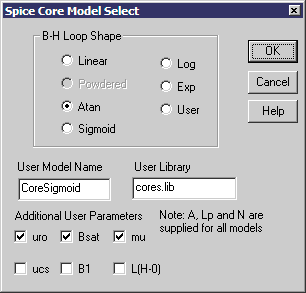If a powdered core model is available, only the Powdered, Exp and User radio buttons will be activated. The update requires a new file serialization scheme to remember these settings, and you won’t be able to use the newly created files with older software. Through this article, the various models will be discussed in depth. For now, Figure 2 illustrates the start-up transient for a peak current-limited flyback design using the Atan model. Figure 1: The new Magnetics designer core selection dialog.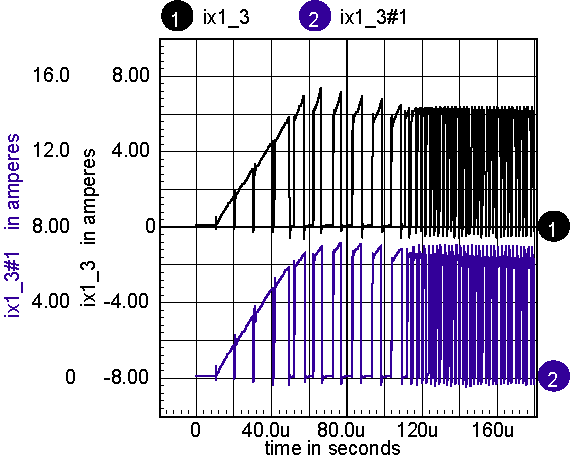Figure 2: Non-linear model shows primary side current, top is non-linear atan model, bottom is a linear core model.
TOP
 Simulation Tips
Before going on to describe construction of saturable core models, here’s a few useful simulation techniques used in deriving the new models. You can get a lot of extra mileage from your simulator by running DC sweeps. Here are a couple of tricks. First, when the data tends to be spaced uniformly in a log plot, then the sweep variable can be expanded using an exponential function. Then when the log is taken, the data point will be spaced uniformly. If you don’t use this trick, you need to set the sweep step very small to resolve the data for larger x-axis values. That causes too much unnecessary data for smaller x-axis values. The sweep generator model in our library does this by setting

.param K2=ln(Imax/Imin)
.param K1=Imax*exp(-K2)
B1 lo hi I={K1}*exp({K2}*v(cntrl))

The sweep variable then ranges from 0 to 1. Example:

.DC V1 0 1 10m

Next, the u-H plot can be obtained by differentiating the B-H plot. The differentiation in the model uses an inductor to differentiate current and feed it back as a voltage. But that process requires time domain or frequency domain analysis. A simple DC sweep with post-simulation differentiation achieves the same objective, and with a lot less computational effort. Figure 3 illustrates the result.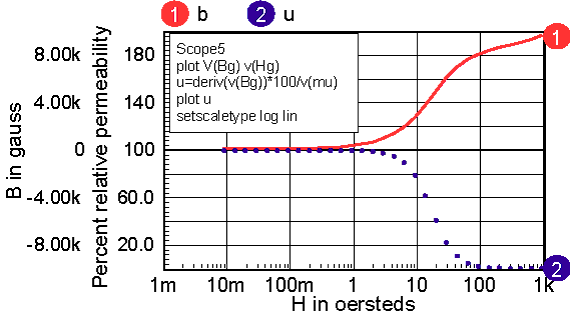Figure 3: The sweep generator spaces data uniformly in a log plot, and the permeability can be calculated by taking the derivative of the B-H plot.
TOP
 Nonlinear Inductor Models
Nonlinear inductance-verses- current characteristics are analogous to the nonlinear capacitor modeling described in NL76. Instead of charge verses voltage, flux verses current is used to control the nonlinearity. The concept of magnetic flux comes from electromagnetic theory and Maxwell’s Equations. These equations can be simplified when the flux is uniform throughout the magnetic path. Here’s what you need to know about magnetic circuits to follow this article (This section can be skipped):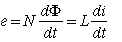1 Where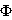is the magnetic flux N is the number of turns L is the inductance i is current t is time
It’s convenient to define the magnetic field,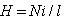And flux density as,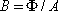, where A is the core cross sectional area andis the magnetic path length.

Ampere’s law, derived from Maxwell’s equations states: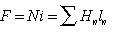1.1

where F is the magneto-motive force and H is the magnetic field. The core consists or n series segments. For a simple core with an air-gap, there are 2 segments consisting of the core and the gap. We’ll get back to the reluctance model after deriving the equation for inductance.

Two common system of units are used, CGS with H in oersteds and B in gauss. The Standard or SI system uses the MKS system and defines H in ampere-turns/meter, and B in teslas (volt-seconds/sq-meter/turn) , where 1 tesla = 1e4 gauss. Substituting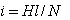and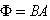, you get the following equation for inductance in SI units: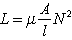2.0 where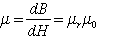2.1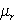is the relative permeability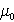is the permeability of free space. In CGS units,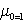,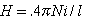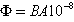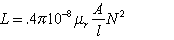2.2 and in SI units,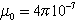,Then, the B-H curves and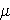-H curves are not dependant on core area or path length; they are just material properties. The CGS system makes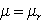because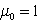so there is still a following that prefers the older CGS system because the integral ofvs. H gives B vs. H without adjusting for units.

For a specific core we can letand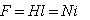. F becomes analogous to voltage in the magnetic circuit andacts like current. Then a new quantity, Reluctance, can be defined by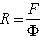. For a simple core,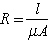, on the other hand, if the core consists of several magnetic paths with different permeability and geometry, the reluctance model can be used like a network of resistors to solve the magnetic equation. The attractive thing about this model is that the network topology looks just like the physical core topology, making the reluctance model an engineering favorite. Here’s what it looks like for a series gap.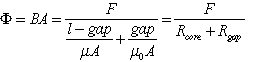3.1 and using equation 1.0, the inductance is given by: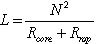SI units 3.2

The model is shown in Figure 4.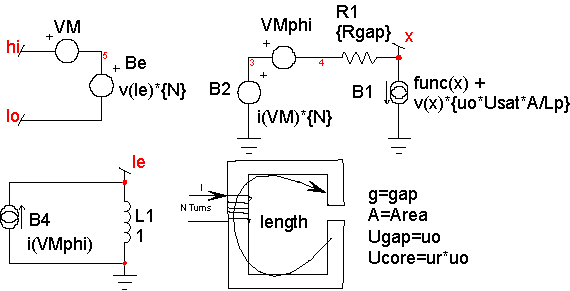Figure 4: The reluctance model includes an air gap. Flux is calculated as I(Vmphi) using a reluctance model, and Faradays equation is implemented using L1 and Be such that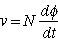Magnetic cores are described by their B-H loop properties. Inductance is proportional to the permeability, which is proportional to the derivative or slope of B with respect to H. A typical B-H curve and permeability-H curve is shown in Figure 5. For most of our discussion, hysteresis will be ignored; it can be shown that the area enclosed by the hysteresis curve is proportional to core loss energy. Moreover, the loss increases significantly at higher frequencies. Placing a resistor, Rcore, across the nonlinear inductor accurately models the high-frequency loss. Hysteresis will appear in the B-H curve if the current used to compute H includes the current delivered to the combination of the resistor and the nonlinear inductor.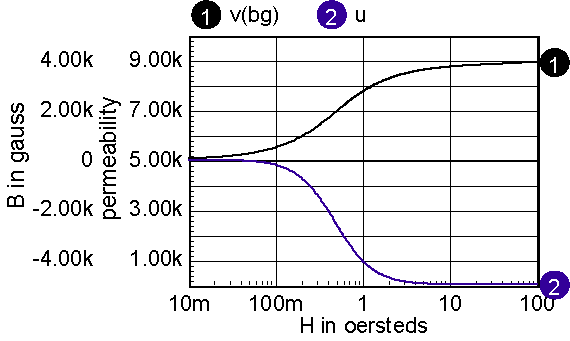Figure 5: A typical B-H curve and its derivative for a ferrite material.

Accurate modeling of inductance requires a good estimate of the permeability-H characteristic. Then the function in the model, func(x) in Figure 4, is formed by integrating the permeability curve.

Inductors are made by using a magnetic material in combination with an air-gap. The gap can be introduced as a discrete gap or as a distributed gap. The most common way of making a distributed gap is by mixing non-magnetic filler with powdered magnetic material. Discrete gaps are made by inserting a dimensionally stable material, such a paper, between the core pieces.

The inductor’s core can be modeled using a table, or by finding continuous functions that approximate the B-H characteristics. Continuous functions are less susceptible to discontinuities, and as a result they should work better in a simulator. When using a table model, the most commonly available data is from a B-H curve. Inaccuracies in transcribing the data from a data sheet curve to a table will result in magnifying the error in the permeability function (that’s because permeability is the derivative of the B-H curve). The B-H curves must be carefully filtered and their derivatives tested to assure a stable simulation. On the other hand, continuous functions can be fit to permeability-H curves using optimization techniques. Errors in transcribing data are reduced statistically, and because the continuous functions tend to describe the device physics. Many data sheets give what appear to be valid continuous functions as the ratio of polynomials. The problem with this approach is that the polynomial ratio departs from physical reality outside the region where data was used to make the best fit. This shortcoming is illustrated in Figure 6. It also illustrates how easy it is to use the IntuScope waveform viewer to evaluate and plot equations.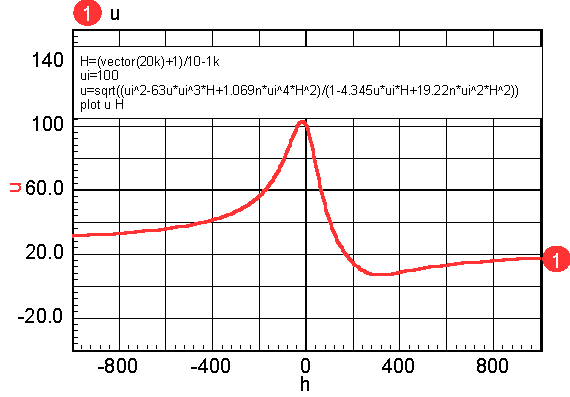Figure 6: The polynomial (taken from a vendor catalog) has odd exponents of H, making it unsymmetrical about H=0. Also, mu increases (incorrectly) beginning at H=316.

Powdered cores exhibit a larger swing in H for the same percentage change in permeability. The reason for this is because the powdered particles will saturate first where they are almost touching each other, slowly increasing the air gap as the magnetic material saturates. Solid cores with a single gap tend to saturate uniformly so that the permeability drops more rapidly. The packing arrangements and shape of the powdered particles vary, based on the manufacturing process. The solution of Maxwell’s equations in a closed form function is unknown, even for simple packing geometry. We will get around this problem by examining several reluctance models based on four generic solutions.

Continuous functions for permeability are useful for defining the-H curves. Many Ferrite curves follow a 1/(1+(mH)^2) curve. This function is integrated as follows: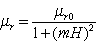4.0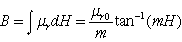4.1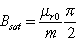4.2 Solving for m and substituting into 4.1 yields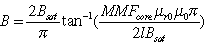4.3 Finally, solving for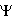and setting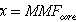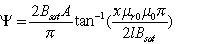4.4

The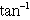(ATAN) function in IsSpice4 has no singularities in itself or its derivatives, so that it’s a safe function to use in behavioral models. The complete atan model is shown in Figure 7.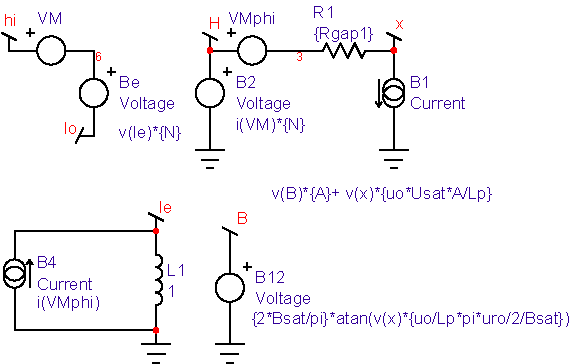Figure 7: Non-linear core with atan B_H shape

Notice that the permeability curves, for example Figure 5, look very much like magnitude vs. frequency plots. When the slope of log(permeability)/log(H) is an integer multiple of 20db/decade, then an even polynomial ratio can be used to describe the curve, and in theory an integral can be found. Note: the restriction on even values arises from the symmetry of permeability for negative MMF-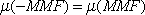. There are 3 more shapes commonly found. First, permeability can be described as:, then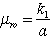5.1

Notice that it retains the even symmetry, but its slope for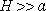goes as 1/H. This property tends to match the characteristics for Kool Mu and High Flux cores. The integral in CGS units yields: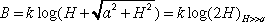5.2

and for H=0,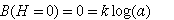5.3

solving for a, we fine a=1, and k1 = uro
Finally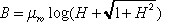5.4

Unlike the atan function, this one grows to infinity. But the slope drops below 1, the permeability of free space. We can therefore define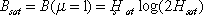as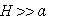5.5

and from 5.1 with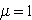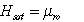Then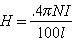with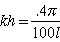where l is in meters, that flips us back to MKS if we also divide K1 by 10k to convert from gauss to teslas. The complete model is shown in Figure 8, where log refers to the B-H shape. Table 1: Bsat, teslas vs uro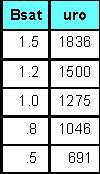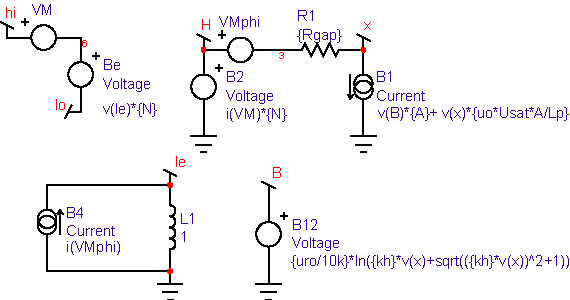Figure 8: The complete corelog model with a log B-H shape

Notice that for all of the models, a Usat term is added in the B1 current (Flux analog) source. Usat is usually set to 1, the relative permeability of free space. It may become convenient to give it a larger value to fit data for very high magnetic fields.

The next shape is the sigmoid B-H curve. It’s extremely sharp and can be used for square-loop material such as Orthonal or Permalloy. Starting from the B-H curve, the sigmoid is defined as: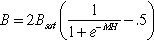6.1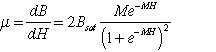6.2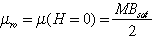6.3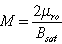6.4

and finally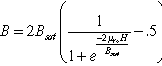6.5

Figure 9 shows the competed model.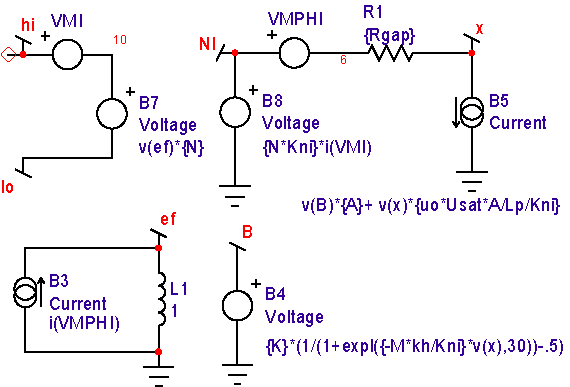Figure 9: Non-linear core with a sigmoid B-H shape

Notice that this model contains a scaling parameter, Kni. It’s used to reduce the voltage that represents the MMF at node NI to normalize the voltage range, so that the VSECTOL convergence option gives good results. It’s usually set to kh, conveniently making the voltage at node NI equal to H in Oersteds.

Yet another B-H loop comes from combining a linear relation below a field,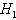, as: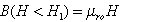Then an exponential function is used thereafter such that the inductance at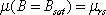. The parameters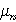,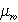,and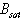define the core as follows.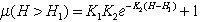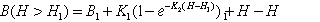where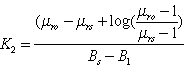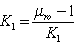This exponential model is used internally in Magnetics Designer for the reluctance model used to compute inductance verses current. It provides a reasonably accurate model for powdered cores; however, there is an abrupt change in the derivative of inductance where the two functions are joined, as seen in Figure 10. It predicts sharper cutoff characteristics for Ferrite cores.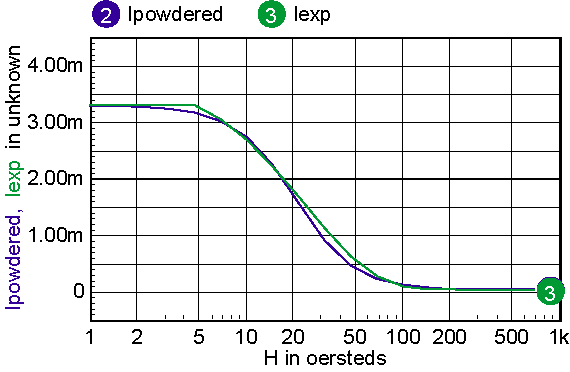Figure 10: Comparing a continuous function, in Lpowdered with the piece-wise-linear function, Lexp, used in Magnetics Designer.

Figure 11 illustrates the differences for a gaped core.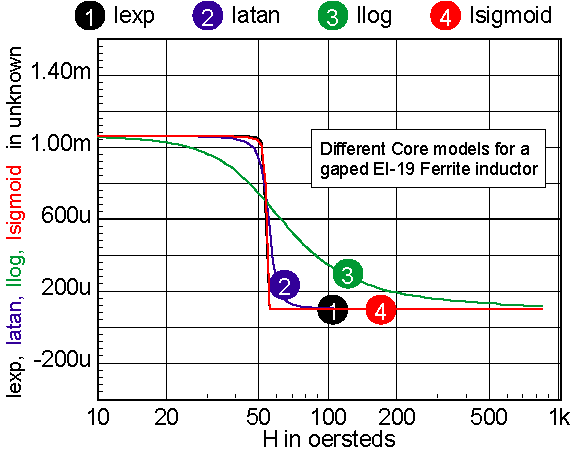Figure 11: Sigmoid and exponential models are nearly identical. Atan and log models have a softer cutoff.

For cores that have distributed gaps, such as MPP or Kool Mu powered cores, a special reluctance model is used. In general, two cores are made in parallel, each with its own gap. The mix parameter tells how much the first core contributes to the H=0 permeability. Note: parallel cores correspond to series inductors.

Table 2 shows the combination of free parameters used to get the best fit to the published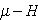data. Free parameters are Model (Log or Atan), Bsat, Usat, uro, ur1, mix. Log cores have no explicit Bsat parameter, and if Mix=1, ur1=1.

Table 2: parameters for powdered cores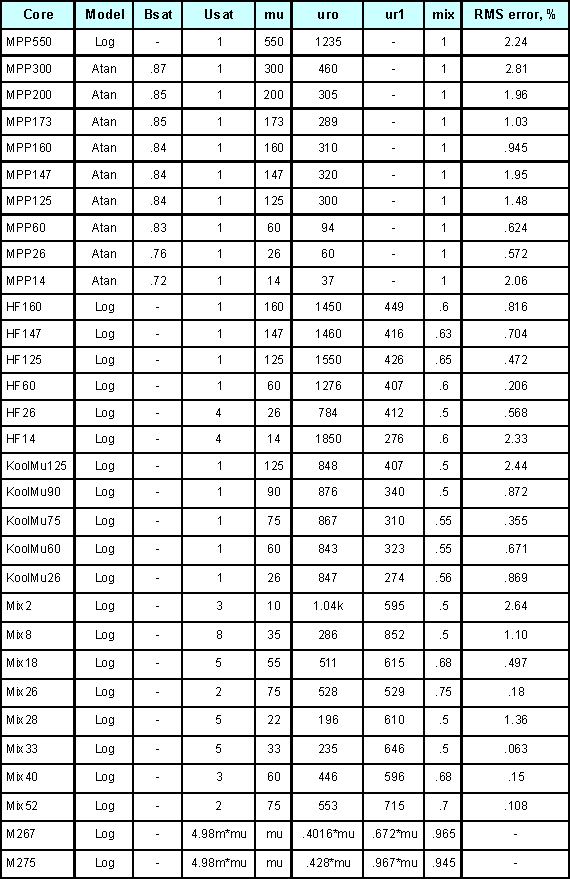The RMS error is in general based on data for percent initial permeability = 80,50,20. When these permeability values can’t be achieved, three different values are substituted. The accuracy to which the charts can be read is larger than the error in the curve fit. Moreover, the initial permeability tolerance is much greater than the curve fit tolerance. Most inaccuracy occurs for percent permeability below 10%, or for deep saturation. Figures 12-16 show the percent permeability verses H for the families in the above table.

It is recommended that the atan model be used for other ferrite cores, and the sigmoid model selected for solid cores. Provisions are made for making your own user models, and connecting them to the Magnetics Designer SPICE model. All of the non-linear models use the IsSpice4 syntax and need to be translated if you use another simulator.

Documentation on how to convert to IsSpice4 syntax is located:
http://www.intusoft.com/convert2isspice4.htm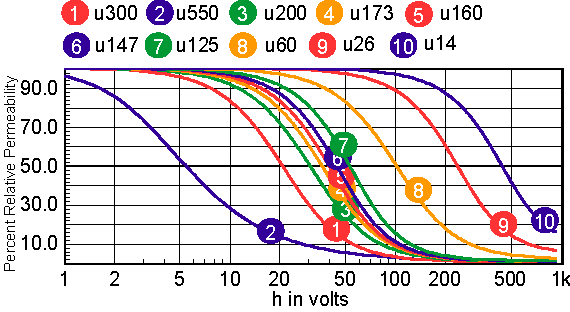Figure 12: MPP curves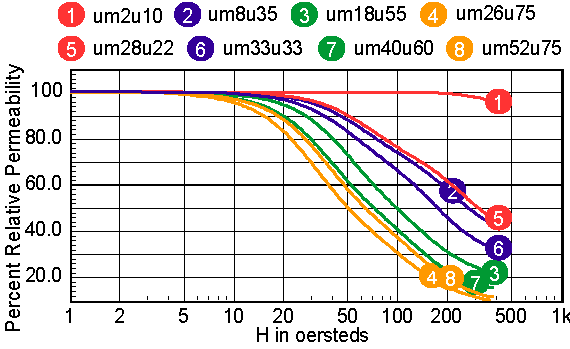Figure 13: Micrometals Mix Family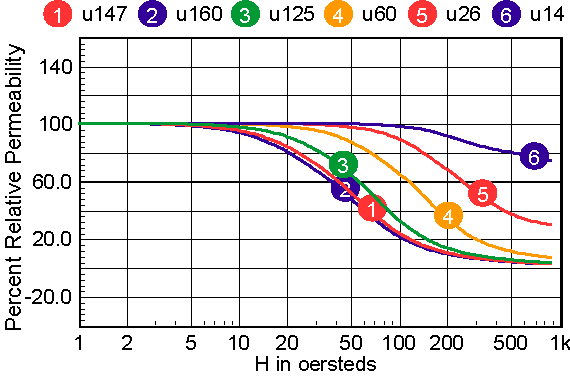Figure 14: The High Flux Family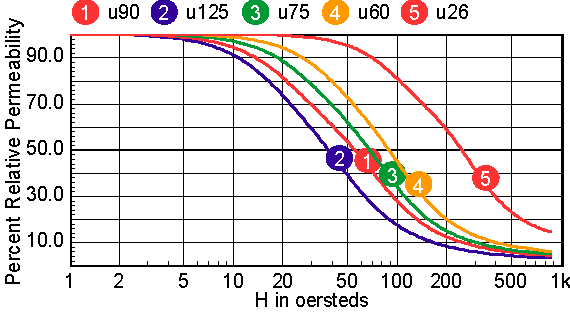Figure 15: The Kool-Mu Family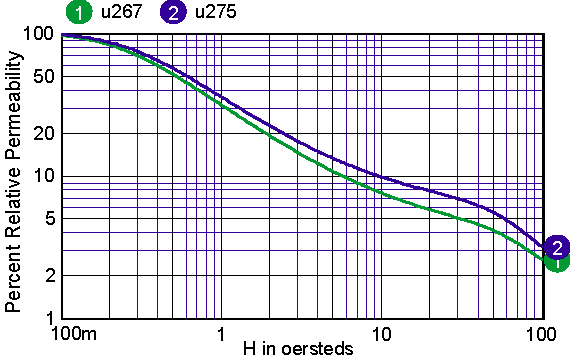Figure 16: Micrometals –267 and –275 characteristics are examples of fitting the log based core models over a permeability range of 50:1.
 Summer Update (Continued)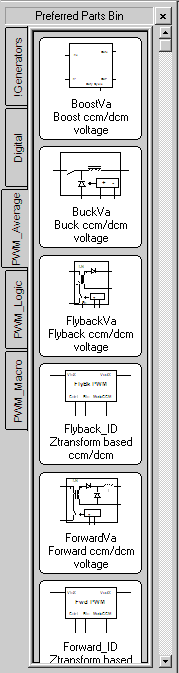Looking further, more detailed error message are supplied to make it easier to debug ICL scripts and B-element expressions. This makes it much easier to figure out what is going on. Another major feature is the new “Preferred Parts Bin,” which has been added to SpiceNet’s parts browser. This dialog-driven feature enables a user to specify from ICAP/4’s 24,000+ simulation models, any number of desired parts, and place them into a custom part bin organized into tabs. The user can now bring up a side panel with a graphical representation of the part, part name, and description. The part can then be dragged and droped from its part bin directly to the schematic. The convergence wizard has been updated to take advantage of Intusoft’s unique simulator options such as vsectol, icstep, and autotol. This unique capability really unlocks the power of IsSpice4. Voltage and current source devices have been enhanced to support expressions without taking derivatives. This means the value of the source is calculated at each iteration, using just the previous state of the simulator. In the DC field, start your expresion with V= or I= to enable this.